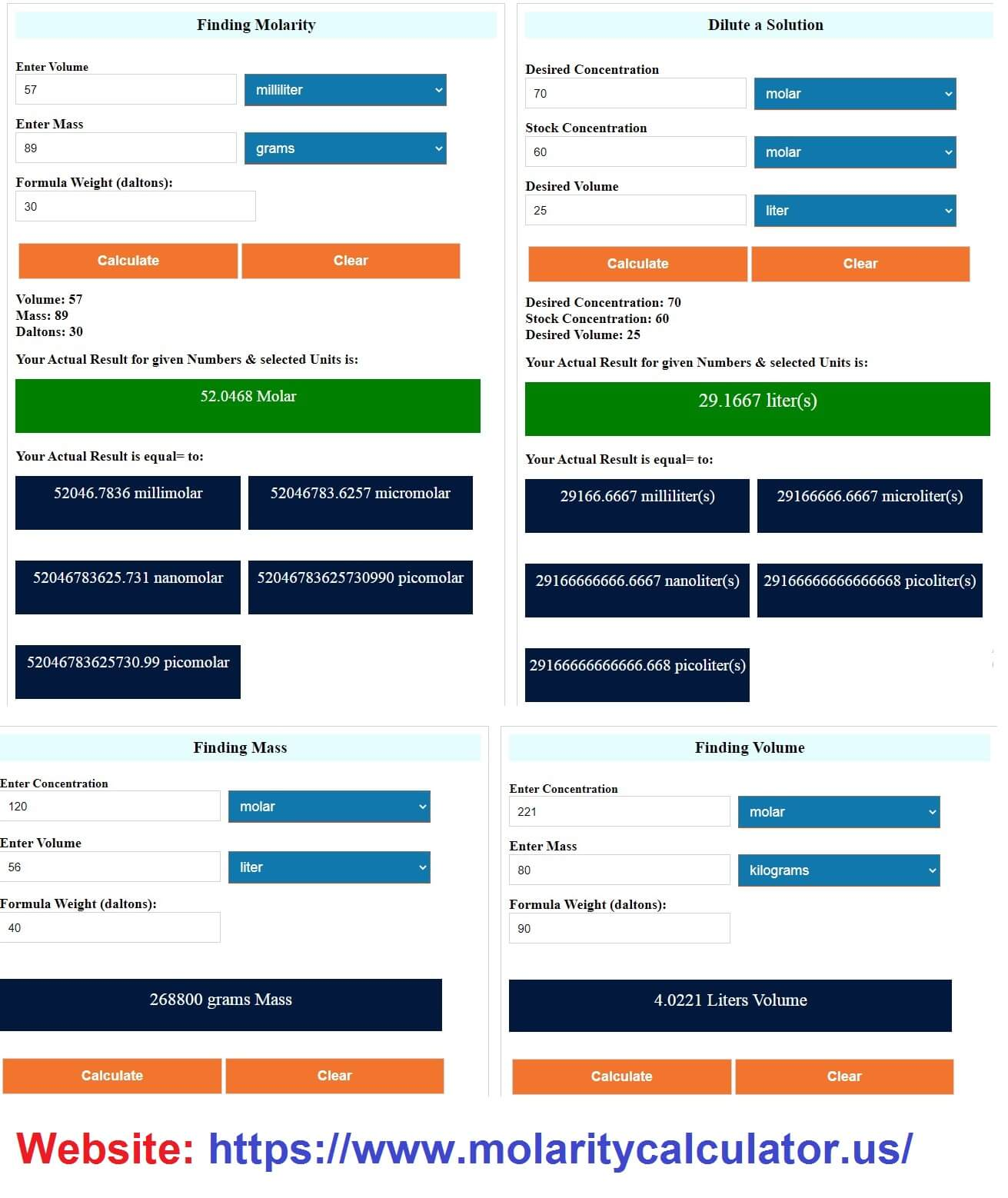# Molarity Calculator

Finding Molarity

Enter Volume

Enter Mass

Formula Weight (daltons):

Dilute a Solution

Desired Concentration

Stock Concentration

Desired Volume

Finding Mass

Enter Concentration

Enter Volume

Formula Weight (daltons):

Finding Volume

Enter Concentration

Enter Mass

Formula Weight (daltons):

## Calculate Molarity

In this section, you will get some guidance about what is molarity? , what is a molar calculator, and how it works? We hope this section becomes efficient for you.

### What is Molarity?

Molarity uses the symbol of (M).
Molarity focuses on the number of moles of solute in a liter (moles/liter) of solution and can be used to calculate the volume of solute.
It is one of the common units of measurement of the concentration of a solution.

Molarity = moles of Solute / liter of solution

What is Molarity Calculator?

It is a tool for calculating the number of moles of solute in a liter (moles/liter), you can give the numbers and select the units (volume, mass, and concentration) to calculate.

How Mass Molarity Calculator works?

There are 4 things (Finding Mass, Finding Volume, Finding Molarity, and Dilute a Solution).
If the values of a unit are not known then you can get the value in its respected section.

Finding Mass How To Calculate Mass

This section is for to find the (Mass) from (Concentration, Volume & Dalton(Atomic Mass or Molecular Weight)). When the (Mass) is not known, you can use this section.
(Enter Value in Concentration textbox and Select the Unit of Concentration)
(Enter Value in Volume textbox and Select the Unit of Volume)
(Enter Value in Dalton (Atomic Mass or Molecular Weight), to Calculate the (Mass).

mass = (concentration * volume) * daltons
exp: (10*15)*50 = 7500 grams of Mass

How To Calculate Volume Finding Volume

This section is for to find the (Volume) from (Concentration, Mass & Dalton(Atomic Mass or Molecular Weight)). When the (Volume) is not known, you can use this section.
(Enter Value in Concentration textbox and Select the Unit of Concentration)
(Enter Value in Mass textbox and Select the Unit of Mass)
(Enter Value in Dalton (Atomic Mass or Molecular Weight), to Calculate the (Volume).

volume = ((mass * 1000) / concentration) / daltons
exp: ((30*1000) / 60) / 15.2 = 32.8947 Liters Volume

#### Finding Molarity How To Calculate Molarity This section is for finding the (Molarity) from (Volume, Mass & Dalton(Atomic Mass or Molecular Weight)). When the (Molarity) is not known, you can use this section. (Enter Value in Volume textbox and Select the Unit of Volume)                 (Enter Value in Mass textbox and Select the Unit of Mass) (Enter Value in Dalton (Atomic Mass or Molecular Weight), to Calculate the (Molarity). In this section we have given multiple results. Check This Tool Too SAT Score Calculator The reason that we include this is that it brings easiness to know the multiple results for a specific number. Suppose you have given some numbers and you get a result in (Molar), so you get the (millimolar, micromolar, nanomolar & picomolar) results also at the same time.   molarity = (mass / (volume * daltons)) * 1000 exp: (22.3 / (15 * 62.6)) * 1000 = 23.7487 Molar

###### Molarity Forumula

molarity = (mass / (volume * daltons)) * 1000 exp: (22.3 / (15 * 62.6)) * 1000 = 23.7487 Molar
mass = (concentration * volume) * daltons exp: (10*15)*50 = 7500 grams of Mass
volume = ((mass * 1000) / concentration) / daltons exp: ((30*1000) / 60) / 15.2 = 32.8947 Liters Volume